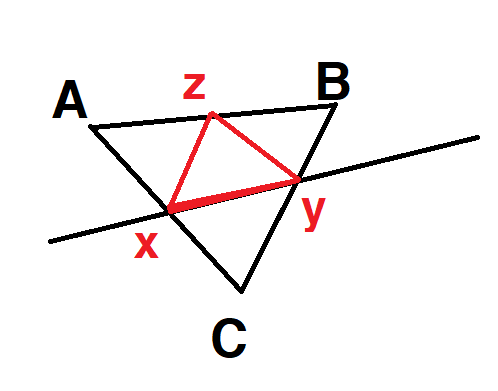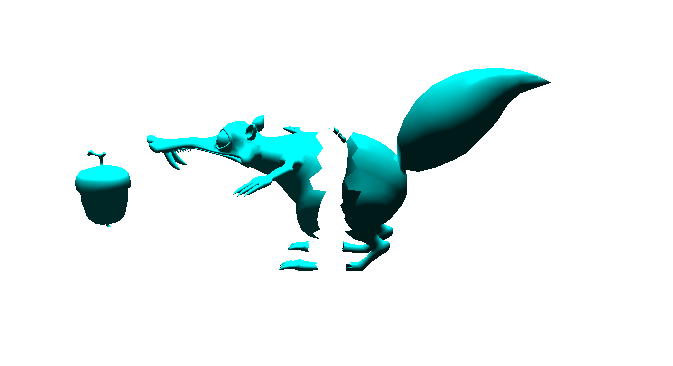# [Newbie][verbose]Plane+Line Intersection

Hello hello Khronos community!
[tl;dr]: need help determining equation of a plane from two crossing lines & finding the point of intersection with a third line
[verbose]:
I’m trying to make an openGL app that’s similar to fruit ninja for an university project. The gist is that I want to cut an arbitrary object in two separate objects by using a slicing plane. I first determine two rays (vec3) that are projected from the camera is looking into the screen where I click. Those two lines determine my slicing plane (a simple plane, no curved surface or anything fancy).
Each vertex has location, normals, uvs and an attribute I added for determining what object it belongs to.

Here’s where the trouble starts.

I have vec3 ray1, ray2 - my projections from the viewport.
I parse each pair of 3 vertices (vec3 A,B,C) that create a triangle and want to determine if AB, BC or AC intersect with the plane (which I don’t know how to calculate the parameters for the equation so I use the normal’s coords - is this ok? ) and then if they intersect - add new vertices to my buffers at the specified intersection points + one point on the middle of the side with two vertices (like in picture below). Points above the plane (on the side of the normal) will remain part of the same object, points below and on will receive another object ID.

It would be of tremendous help if you could tell me how to find the four components of the plane equation and then how to determine the intersection points.

This post is my last resort. I feel incredibly dumb for not understanding how to do geometry in OpenGL :\ I’ve looked at other posts and still don’t get it… I can tell if a point is below or above the plane by doing the dot product with the normal to the plane (which I found to be the cross product between ray1 and ray2) - but representing lines and planes in OpenGL escapes my comprehension.
In any case, thank you for hearing my plea and reading this far.First question: do you want to perform this calculation in object space, eye space or clip space? It doesn’t necessarily matter which you use, but all points and vectors must be in the same space.

A plane is normally defined by an implicit equation of the form:
kx*x+ky*y+kz*z+kw=0
which can be more compactly represented as a dot product
K·X=0
where
K=<kx,ky,kz,kw>
X=<x,y,z,1>
If you have two vectors v1,v2 parallel to the plane and a point p on the plane, then the normal vector n=<kx,ky,kz> is the cross product v1×v2. Given that p lies on the plane, you have n·p+kw=0 => kw=-n·p, i.e. the negation of the dot product between the point p and the normal n. If you perform the calculation in eye space, p is the origin <0,0,0> and thus kw=0. So you don’t need the fourth component in either K or X.

To test whether the plane intersects a triangle , you just need to calculate K·X for each of the triangle’s vertices. If the sign is the same for all three, all lie on the same side of the plane. If the signs are a mix of positive and negative, the plane intersects the triangle.

As for finding the point of intersection: if vertices X1 and X2 lie on opposite sides of the plane, then some interpolated point X=a.X1+b.X2 (with a+b=1, a>0, b>0) lies on the plane.
K·X=0
=> K·(a.X1+b.X2)=0
=> a.K·X1+b.K·X2=0
=> a.(K·X1)=-b.(K·X2)
=> a/b=-(K·X2)/(K·X1)
=> a=(K·X2)/(K·X2-K·X1), b=-(K·X1)/(K·X2-K·X1).
=> a=a’/(a’+b’), b=b’/(a’+b’) where a’=K·X2, b’=-K·X1

1 Like

Since I obtain my rays like in image below, I would assume I should be working with eye space (?) - but then I should also multiply each point I’m checking with the modelViewProjection matrices, right ? I am doing this outside shaders and am accessing the vertices buffers with simple c++. I do this because I need to alter those buffers by inserting new points dynamically - which I don’t know if would be easily done with glsl in one of the shaders (maybe the geometry one :-? )

A few things stand out:

1. You shouldn’t be setting ray_eye.z to 1.0. You can set ray_eye.w to whatever you want as it only changes the length of the vector, not its direction. What matters is the ratios x/z and y/z.
2. If you’re transforming the rays to world space, you also need to transform the viewpoint (<0,0,0,1> in eye space, <0,0,k,0> in clip space) to get the W component of the plane equation. Otherwise the plane will go through the world-space origin.
3. You don’t actually need to transform the vector by each matrix individually. You can transform NDC to object space in one step with inverse(projectionviewmodel)ray_clip. You can even fold in the viewport transformation: inverse(viewportprojectionviewmodel)*ray_clip.

You’re transforming them to world space. If you have a model matrix, you need to transform by the inverse model-view matrix (inverse(view*model)) rather than just the inverse view matrix to get object space. If you’re manipulating the geometry on the CPU, you’ll want the planes in object space to match the vertices.

Thank you for taking the time to explain these things to me, I am very grateful for finding this community.
I’ll update tomorrow if I get my code to do what’s intended.

Well, it’s not much at the moment but I have had some success with separating the object by the simple case of all 3 points above or below (which doesn’t involve adding extra points). The cuts look jagged and the inside of the model is empty.

I am using glDrawArrays(GL_TRIANGLES, 0, vertices.size()); currently and introducing extra points that don’t screw up the model is proving to be a pain in the ass, but I think I could use GL_TRIANGLE_STRIP somehow to add an extra point and patch up the empty parts after the cut.If you cut a triangle, you typically end up with three triangles. If the triangle straddles the plane, you have one vertex on one side of the plane and two on the other. The single vertex (along with the two new vertices generated by the cut) gives a triangle, the two vertices give a quadrilateral (which is usually split into two triangles).

If you want to close the surface, first you’ll need to identify the distinct paths; e.g. in the above image, there’s one path around the body and one around each foot (and maybe something else; the right elbow? hard to see). Each path will form a polygon (which typically won’t be convex); you’ll need to tessellate it into triangles. Or you could use stencil operations (but this will fail quite hard if the original geometry isn’t clean). Neither approach is simple enough to describe in this post. If you take the stencilling approach, you might just want to use a user-defined clip plane (glClipPlane() etc in legacy OpenGL, gl_ClipDistance with vertex shaders) rather than calculating intersections.

1 Like

My last remaining problem: the rays I cast aren’t properly obtained -> the cutting planes are 95% centered on vertical cuts and at the very bottom when doing horizontal cuts. Can you shed some light on the mess I’m making below?

I decided that the best approach would be to make the cuts in object space so that I don’t have to transform each point by the projection matrix (since I work on the CPU and access the unprocessed object data anyway).

In my vertex shader I transform the points like this:
gl_Position = modelViewProjectionMatrix * vec4(vPos, 1.0); // model * view * projection * vPos

How are you generating the plane?

`getObjectSpaceRay()` returns a point in object space. That point will be a fixed distance in front of the viewpoint. If you want a direction vector, you need to subtract the object-space viewpoint (i.e. `cameraPos`) from the result.

1 Like

I was using the result as a direction vector and then with two direction vectors I was doing a cross product to determine the plane normal. With that I would then check if points are below, on or above using the dot product.

I’ve tried subtracting the cameraPos from the point before returning it, but that doesn’t seem to help in regards to the accuracy of the cuts.

Once you’ve obtained the plane normal, you need to take the dot product of the normal with the viewpoint to obtain the offset. Otherwise the cutting plane will go through the object-space origin.

IOW, the plane is the set of points P such that dot(normal,P)=dot(normal,viewpoint) => dot(normal,P)-dot(normal,viewpoint)=0.

1 Like